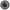Java - Online Test - Abstract classes - javasearch.buggybread.com## Search Java Test Questions223 test questions in repository.

Java - Test Questions on 'Abstract classes' - 2 questions foundIf X implements Y and Y extends Z, Which of the following initialization is correct ? Core Java
X x = new Y();
X x = new Z();
Z z = new Y();
Z z = new X();interfacesIf class A implements X interface, and Class B implements Y interface, and X is the parent of Y, Which of the following initialization is correct ? Core Java
A a = new B();
Y y = new A();
X x = new B();
Y y = new A();interfaces   inheritance

comments powered by Disqus